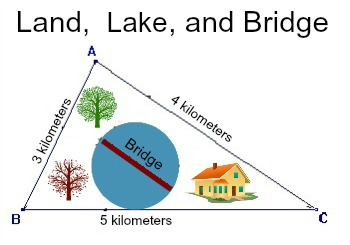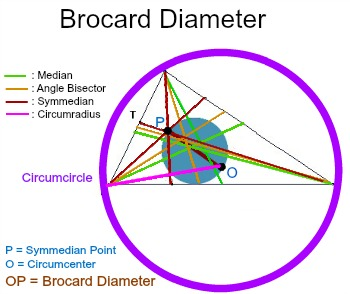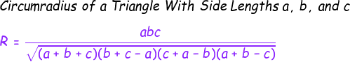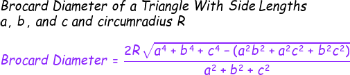# Brocard Diameter: Definition & Formula

Instructor: Laura Pennington

Laura received her Master's degree in Pure Mathematics from Michigan State University, and her Bachelor's degree in Mathematics from Grand Valley State University. She has 20 years of experience teaching collegiate mathematics at various institutions.

Brocard diameters are a really neat characteristic that triangles possess. This lesson will define the Brocard diameter of a triangle. We will also look at how to find its length, and do so in a real world application.

## Brocard Diameter

Suppose that a builder buys a large triangular plot of land, with side lengths of 3 kilometers, 4 kilometers, and 5 kilometers, to build his dream house on. On the land in the back of the house, there is a fairly large circular lake that the builder would like to build a bridge across as shown in the image.As it turns out, this bridge actually represents a really neat characteristic that every triangle possesses called the Brocard diameter. In order to define the Brocard diameter of a triangle, we are going to need to be familiar with quite a few other definitions, so bear with me here as we list some vocabulary.

• Median: The median of a triangle is a line segment that runs from a vertex of the triangle to the midpoint of the side opposite that vertex.
• Angle bisector: A line that cuts the angle at any vertex of a triangle exactly in half.
• Symmedian: The three symmedians of a triangle are the lines that are reflections of the medians of the triangle over their corresponding angle bisector.
• Symmedian Point: The symmedian point of a triangle is the point where the triangle's three symmedians intersect.
• Circumcircle: The circumcircle of a triangle is a circle drawn around the triangle that passes through all of the triangle's vertices.
• Circumcenter: The circumcenter of a triangle is the center point of the triangle's circumcircle.
• Circumradius: The circumradius of a triangle is the radius of the triangle's circumcircle.
• Brocard Circle: The Brocard circle of a triangle is the circle that passes through the triangle's symmedian point and the triangle's circumcenter.

Geesh! This is turning into a mathematical dictionary! Who knew there were so many aspects to a simple triangle? Well, the good news is that now we have everything we need to define the Brocard diameter of a triangle!

The Brocard diameter of a triangle is the diameter of the triangle's Brocard circle that runs from the symmedian point of the triangle to the circumcenter of the triangle.Ah-ha! We can see now that the lake is the Brocard circle of the triangular plot of land, and the bridge that the builder would like to build across the lake is the Brocard diameter of the triangular plot of land.

## Length of a Brocard Diameter

Since the bridge is across water, the builder is having a hard time determining how long the bridge needs to be. Thankfully, we have a couple of formulas that will help him out! You see, we have a nice formula for the length of the Brocard diameter of a triangle based on the side lengths of the triangle. This formula is also based on the length of the circumradius of the triangle, so first, let's look at the formula for the length of the circumradius of a triangle.Now that we know the formula for the length of the circumradius of a triangle with side lengths a, b, and c, we can learn the formula for the length of the Brocard diameter of a triangle with side lengths a, b, c, and circumradius of length R.Great! So to find the length of the Brocard diameter of a triangle with side lengths a, b, and c, we use the following steps:

1. Plug a, b, and c into the formula for the circumradius, R, of the triangle, and calculate the circumradius.
2. Plug a, b, c, and R into the formula for the length of the Brocard diameter of the triangle and simplify to get the Brocard diameter.

Let's give it a go with finding the length of this bridge so that the builder can get to work!

## Example

To find the length of the bridge, we are finding the length of the Brocard diameter of the triangular plot of land, with side lengths 3 kilometers, 4 kilometers, and 5 kilometers, so we can just take it through our steps.

To unlock this lesson you must be a Study.com Member.

### Register to view this lesson

Are you a student or a teacher?

Back

### Resources created by teachers for teachers

Over 30,000 video lessons & teaching resources‐all in one place.Video lessonsQuizzes & WorksheetsClassroom IntegrationLesson Plans

I would definitely recommend Study.com to my colleagues. It’s like a teacher waved a magic wand and did the work for me. I feel like it’s a lifeline.

Jennifer B.
TeacherCreate an account to start this course today
Used by over 30 million students worldwide